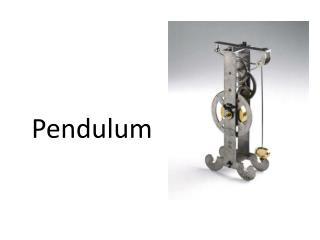DownloadDownload PresentationPendulum

# Pendulum

Télécharger la présentation## Pendulum

- - - - - - - - - - - - - - - - - - - - - - - - - - - E N D - - - - - - - - - - - - - - - - - - - - - - - - - - -
##### Presentation Transcript

1. Pendulum

2. the length of the pendulum is measured from the pendulum's point of suspension to the center of mass of its bob. Its amplitude is the string's angular displacement from its vertical or its equilibrium position.

3. The period is the time in which a pendulum completes a single oscillation, i.e., returns to the position it was in at the beginning of the period. • For example: The time required for the pendulum to move from its most extreme right position back to that point. The pendulum passes twice through the arc during each period. Where L is the length of the pendulum in meters g is the gravitational field strength, or acceleration due to gravity • T, the period of a simple pendulum depends on the length of the string, • the mass of the pendulum bob however does not affect the amplitude of the swing